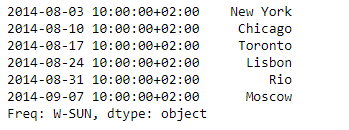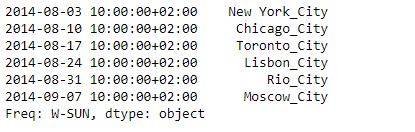# Python | Pandas Series.transform()

Pandas series is a One-dimensional ndarray with axis labels. The labels need not be unique but must be a hashable type. The object supports both integer- and label-based indexing and provides a host of methods for performing operations involving the index.

Pandas` Series.transform()` function Call func (the passed function) on self producing a Series with transformed values and that has the same axis length as self.

Syntax: Series.transform(func, axis=0, *args, **kwargs)

Parameter :
func : If a function, must either work when passed a Series or when passed to Series.apply
axis : Parameter needed for compatibility with DataFrame.
*args : Positional arguments to pass to func.
**kwargs : Keyword arguments to pass to func.

Returns : Returns series that must have the same length as self.

Example #1: Use `Series.transform()` function to transform the elements of the given Series object. Append ‘_City’ at the end of each city name.

 `# importing pandas as pd ` `import` `pandas as pd ` ` `  `# Creating the Series ` `sr ``=` `pd.Series([``'New York'``, ``'Chicago'``, ``'Toronto'``, ``'Lisbon'``, ``'Rio'``, ``'Moscow'``]) ` ` `  `# Create the Datetime Index ` `didx ``=` `pd.DatetimeIndex(start ``=``'2014-08-01 10:00'``, freq ``=``'W'``,  ` `                     ``periods ``=` `6``, tz ``=` `'Europe/Berlin'``)  ` ` `  `# set the index ` `sr.index ``=` `didx ` ` `  `# Print the series ` `print``(sr) `

Output :Now we will use `Series.transform()` function to append ‘_City’ at the end of each city name.

 `# append '_City' ` `sr.transform(``lambda` `x : x ``+` `'_City'``) `

Output :As we can see in the output, the `Series.transform()` function has successfully appended the desired keyword at the end of which city name.

Example #2: Use `Dataframe.transform()` function to transform the data of the given Dataframe. Increase the ticket cost of each even by 1000.

 `# importing pandas as pd ` `import` `pandas as pd ` ` `  `# Creating the Dataframe ` `df ``=` `pd.DataFrame({``'Date'``:[``'10/2/2011'``, ``'11/2/2011'``, ``'12/2/2011'``, ``'13/2/2011'``], ` `                    ``'Event'``:[``'Music'``, ``'Poetry'``, ``'Theatre'``, ``'Comedy'``], ` `                    ``'Cost'``:[``10000``, ``5000``, ``15000``, ``2000``]}) ` ` `  `# Print the dataframe ` `print``(df) `

Output :Now we will use `Dataframe.transform()` function to increase the ticket cost by 1000

 `# transform the 'Cost' column ` `df[``'Cost'``] ``=` `df[``'Cost'``].transform(``lambda` `x : x ``+` `1000``) ` ` `  `# Print the dataframe after modification ` `print``(df) `

Output :As we can see in the output, the `Dataframe.transform()` function has successfully increased the ticket cost of each event by 1000.

My Personal Notes arrow_drop_upCheck out this Author's contributed articles.

If you like GeeksforGeeks and would like to contribute, you can also write an article using contribute.geeksforgeeks.org or mail your article to contribute@geeksforgeeks.org. See your article appearing on the GeeksforGeeks main page and help other Geeks.

Please Improve this article if you find anything incorrect by clicking on the "Improve Article" button below.

Article Tags :

Be the First to upvote.

Please write to us at contribute@geeksforgeeks.org to report any issue with the above content.